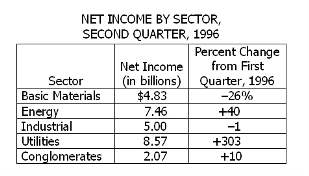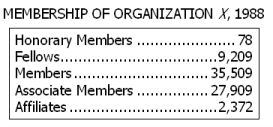# Problem Solving Sample Questions

## Problem Solving Sample Questions | 7 Questions

1. Dick and Jane each saved \$3,000 in 1989. In 1990 Dick saved 8 percent more than in 1989, and together he and Jane saved a total of \$5,000. Approximately what percent less did Jane save in 1990 than in 1989?

A. 8%
B. 25%
C. 41%
D. 59%
E. 70%

Answer Explanation: In 1990 Dick saved 8 percent more than the \$3,000 he saved in 1989, which amounted to (1.08)(\$3,000), or \$3,240. In 1990 he and Jane together saved \$5,000. Thus, Jane must have saved only \$5,000 - \$3,240 = \$1,760, which is \$1,240 less than she saved in 1990. Therefore, in 1990 Jane saved approximately \$1,240/\$3,000 = 41% less than she saved in 1989. The best answer is C.

2. The table below represents the combined net income of all United States companies in each of five sectors for the second quarter of 1996. Which sector had the greatest net income during the first quarter of 1996?A. Basic Materials
B. Energy
C. Industrial
D. Utilities
E. Conglomerates

Answer Explanation: The table shows net income in billions of dollars and the percent change from the first quarter to the second. According to the table, net income in the basic materials sector decreased by 26 percent from the first quarter to the second. If x represents the net income in the first quarter, (0.74)x = 4.83, and x = 6.53. The energy sector net income increased by 40 percent. If y represents the net income in the first quarter, (1.40)y = 7.46, so y < 6. The industrial sector changed by only 1 percent to 5.00, so the first quarter value had to be less than 6.53. For utilities, the net income increased about 300 percent, which means that the net income in the first quarter was about 1/4 of 8.57, which is clearly less than 6.53. The conglomerates sector net income the first quarter was less than 2.07, so the basic materials net income was greatest in the first quarter. The best answer is A.

3. According to the table below, the number of fellows was approximately what percent of the total membership of Organization X ?A. 9%
B. 12%
C. 18%
D. 25%
E. 35%

Answer Explanation: From the table, the number of fellows is 9,209, and the total membership is the sum of the 5 numbers, which is 75,077. Therefore, the number of fellows is 9,209/75,077 of the total membership, or approximately 12 percent. The best answer is B.

4. The regular price per can of a certain brand of soda is \$0.40. If the regular price per can is discounted 15 percent when the soda is purchased in 24-can cases, what is the price of 72 cans of this brand of soda purchased in 24-can cases?

A. \$16.32
B. \$18.00
C. \$21.60
D. \$24.48
E. \$28.80

Answer Explanation: The discounted price of one can of soda is (0.85)(\$0.40), or \$0.34. Therefore, the price of 72 cans of soda at the discounted price would be (72)(\$0.34), or \$24.48. The best answer is D.

5. Increasing the original price of an article by 15 percent and then increasing the new price by 15 percent is equivalent to increasing the original price by:

A. 32.25%
B. 31.00%
C. 30.25%
D. 30.00%
E. 22.50%

If p is the original price, then the 15 percent increase in price results in a price of 1.15p. The next 15 percent increase in price results in a price of 1.15(1.15p), or 1.3225p. Thus, the price increased by 1.3225p – p = 0.3225p, or 32.25% of p. The best answer is A.

6. One hour after Yolanda started walking from X to Y, a distance of 45 miles, Bob started walking along the same road from Y to X. If Yolanda’s walking rate was 3 miles per hour and Bob’s was 4 miles per hour, how many miles had Bob walked when they met?

A. 24
B. 23
C. 22
D. 21
E. 19.5

Answer Explanation: Let t be the number of hours that Bob had walked when he met Yolanda. Then, when they met, Bob had walked 4t miles and Yolanda had walked 3(t + 1) miles. These distances must sum to 45 miles, so 4t + 3(t + 1) = 45, which may be solved for t as follows:

4t + 3(t + 1) = 45
4t + 3t + 3 = 45
7t = 42
T = 6 (hours)

Therefore, Bob had walked 4t = 4(6) = 24 miles when they met. The best answer is A.

7. If the population of a certain country increases at the rate of one person every 15 seconds, by how many persons does the population increase in 20 minutes?